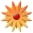A Math Twist

Page Views: 4393

Email This Lesson Plan to Me
Overall Rating:(5.0 stars, 1 ratings)

 Keywords: math, computers, movies Subject(s): Video, Technology, Writing, Photography, Math Grades 3 through 6 School: Holly Oak Elementary School, San Jose, CA Planned By: Bryan Feci Original Author: Bryan Feci, San Jose
Imagine what it would be like if students complete class assignments using computers rather than paper and pencil tasks. Here is how this scenario is possible:
Fourth graders need to be able to multiply multiple-digit numbers (i.e. 6,324x49) and divide multiple-digit numbers by single digits (i.e. 4,329/7). Therefore, my students will learn multiplication and division using various software programs on our current classroom computers. Having an LCD projector, I am able to demonstrate both the mathematical procedures and creatively Microsoft Word to extend learning.
Microsoft Word has the potential for students to practice mathematical procedures for solving multiplication and division problems. As I have done in previous years, students work on Word templates I create to solve math problems (including word problems). Text boxes are created for each number in a math problem. Students solve various math problems on these templates. The �drawing� toolbar in Microsoft Word allows students to construct the lines and symbols needed for creating multiplication and division problems identical to what is normally written down on binder paper. In the past, I have used various math templates to differentiate for at-risk and advanced students since one size does not fit all in learning.
A variation of this would be to use movie making software allowing students to narrate a demonstration how to solve various math problems rather than having everything on paper.
This project can be adjusted for many math standards. The fourth grade math and language arts standards taught in this project include: NS3.3 (Solve problems involving multiplication of multidigit numbers by two-digit numbers), NS3.4 (Solve problems involving division of multidigit numbers by one-digit numbers), MR1.2 (Determine when and how to break a problem into simpler parts), MR2.3 (Use a variety of methods�to explain mathematical reasoning), MR2.4 (Express the solution clearly using appropriate mathematical notation, terms, and clear language), LS1.4 (Give precise directions and instructions), and LS1.6 (Use traditional structures for conveying information).
Evaluation:
Student assignments will be evaluated using rubric scores from 1 to 4. Students will earn points based on the following criteria: 1) accuracy of calculations and whether or not students show their work with regrouping when necessary, 2) accuracy of step by step instructions for solving the math problem, 3) complexity of problem solved, 4) how effective student uses textboxes, lines, arrows, word art, clip art, borders in their problems, 5) template used (whether created from scratch, continued one started for them, or a fill-in template), and 6) how well student explains how to solve the math problem in complete sentences using the document camera.

 Materials: Mobile Labs, Elementary